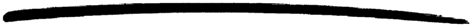# ordsets

ordsets

### MODULE SUMMARY

Functions for Manipulating Sets as Ordered Lists

### DESCRIPTION

Sets are collections of elements with no duplicate elements. An ordset is a representation of a set, where an ordered list is used to store the elements of the set. An ordered list is more efficient than an unordered list.

This module provides exactly the same interface as the module sets but with a defined representation. One difference is that while sets considers two elements as different if they do not match (=:=), this module considers two elements as different if and only if they do not compare equal (==).

### DATA TYPES

```ordered_set()
as returned by new/0
```

### EXPORTS

Types:

Ordset = ordered_set()

Returns a new empty ordered set.

Types:

Ordset = term()

Returns true if Ordset is an ordered set of elements, otherwise false.

Types:

Ordset = term()

Returns the number of elements in Ordset.

Types:

Ordset = ordered_set()
List = [term()]

Returns the elements of Ordset as a list.

Types:

List = [term()]
Ordset = ordered_set()

Returns an ordered set of the elements in List.

Types:

Element = term()
Ordset = ordered_set()

Returns true if Element is an element of Ordset, otherwise false.

Types:

Element = term()
Ordset1 = Ordset2 = ordered_set()

Returns a new ordered set formed from Ordset1 with Element inserted.

Types:

Element = term()
Ordset1 = Ordset2 = ordered_set()

Returns Ordset1, but with Element removed.

Types:

Ordset1 = Ordset2 = Ordset3 = ordered_set()

Returns the merged (union) set of Ordset1 and Ordset2.

Types:

OrdsetList = [ordered_set()]
Ordset = ordered_set()

Returns the merged (union) set of the list of sets.

Types:

Ordset1 = Ordset2 = Ordset3 = ordered_set()

Returns the intersection of Ordset1 and Ordset2.

Types:

OrdsetList = [ordered_set()]
Ordset = ordered_set()

Returns the intersection of the non-empty list of sets.

Types:

Ordset1 = Ordset2 = ordered_set()

Returns true if Ordset1 and Ordset2 are disjoint (have no elements in common), and false otherwise.

Types:

Ordset1 = Ordset2 = Ordset3 = ordered_set()

Returns only the elements of Ordset1 which are not also elements of Ordset2.

Types:

Ordset1 = Ordset2 = ordered_set()

Returns true when every element of Ordset1 is also a member of Ordset2, otherwise false.

Types:

Function = fun (E, AccIn) -> AccOut
Acc0 = Acc1 = AccIn = AccOut = term()
Ordset = ordered_set()

Fold Function over every element in Ordset returning the final value of the accumulator.

Types:

Pred = fun (E) -> bool()
Set1 = Set2 = ordered_set()

Filter elements in Set1 with boolean function Fun.